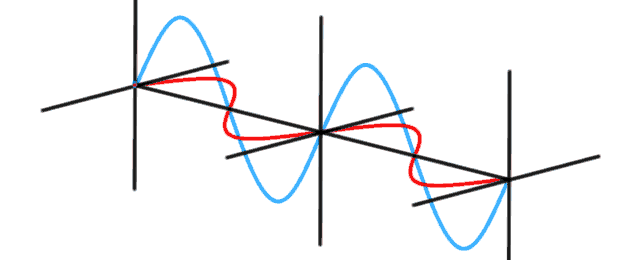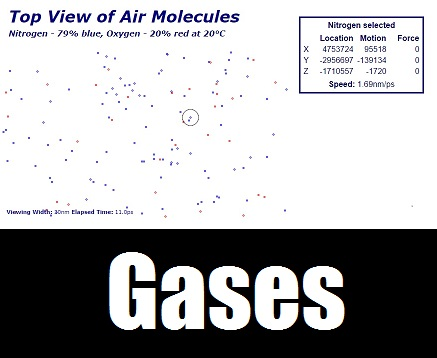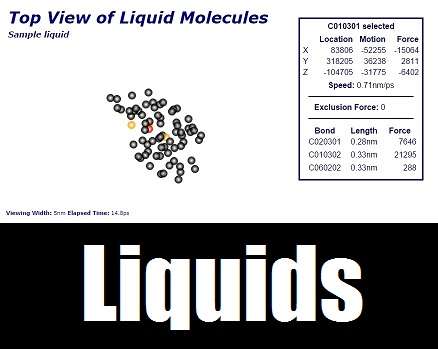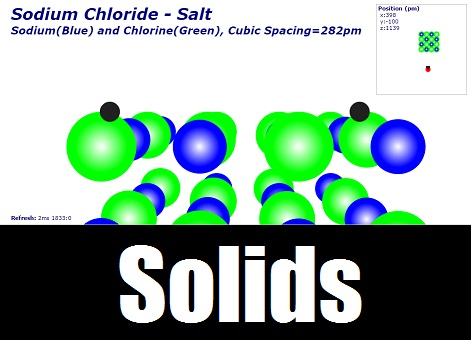#### PhotonsModelling photon phase and polarization. Animations for energy storage, trapped in a cavity and an MM interferometer.

Next Animation Step:

• Photon shotgun pattern for diffraction around a slit (laser past a needle);
• Photon shotgun pattern for diffraction through a pinhole;
• Bell style experiment - animation of violating Bell inequality;
• Create a frequence "pulse";

#### Hydrogen Energy States

3D rigid rotator on a sphere is used to calculate the Hydrogen energy levels quantum mechanically to get the quantum numbers, examples:

Next Animation Step:

Rigid rotator expanded with in/out wave function to calculate levels n.

#### States of MatterModelling Gases: A box 30 x 10 x 7.5 nanometers (10^-9), gridspacing in femtometers (10^-15), timespacing in 10's of femtoseconds (10^-15).

Next Calculation Step:

• Find how many molecules to expect at different temperatures and their average velocity, ie. 10 or 30 degrees.
• Find how many molecules and their average velocity of helium to expect in this volume at 20 degrees degrees.

Next Animation Step:

• Collisions between molecules causing reactions involving break and forming bonds based on modeling bonding structure as here.Modelling Liquids: A box 5 x 3 x 2 nanometers (10^-9), gridspacing in femtometers (10^-15), timespacing in 10's of femtoseconds (10^-15).

Next Calculation Step:

• Find the average length, number of bonds and strength of different bonds in water.
• Find the average length, number of bonds and strength of different bonds in gasoline.

Next Animation Step:

• Break link if stretched to far, relink if unlinked molecules close - surface tension should form;
• Let different numbers of bonds settle into crystal structures;
• Add secondary atoms - ie. hydrogen to an oxygen or carbon grid;Modelling solids: Walk around a solid and see your two anntena 100 picometers (10^-12) ahead of you, separated by 100 picometers.

Next Calculation Step:

• Calculate grid spacing for Simple, Body-centered and Face-centered cubic.

Next Animation Step:

• Let different crystal structures;
• Add electron grid and attract electrons;
• Model of a Scanning Tunneling Microscope (STM);

## Bringing the world to life

Step by step animations through a Euclidian landscape.

## Photons are spin 1

Spin 1 particles have three different and unique properties.

* They can cause something they hit to spin right (this photon would be spin+1)
* spin left (This one would be spin-1) or
* not spin at all (spin 0 state).

Photons can have a particular angle where there axis of spin is most likely to be seen (spin 0). Photons can also have their axis of spin spinning to the right (spin+1) or to the left (spin-1).